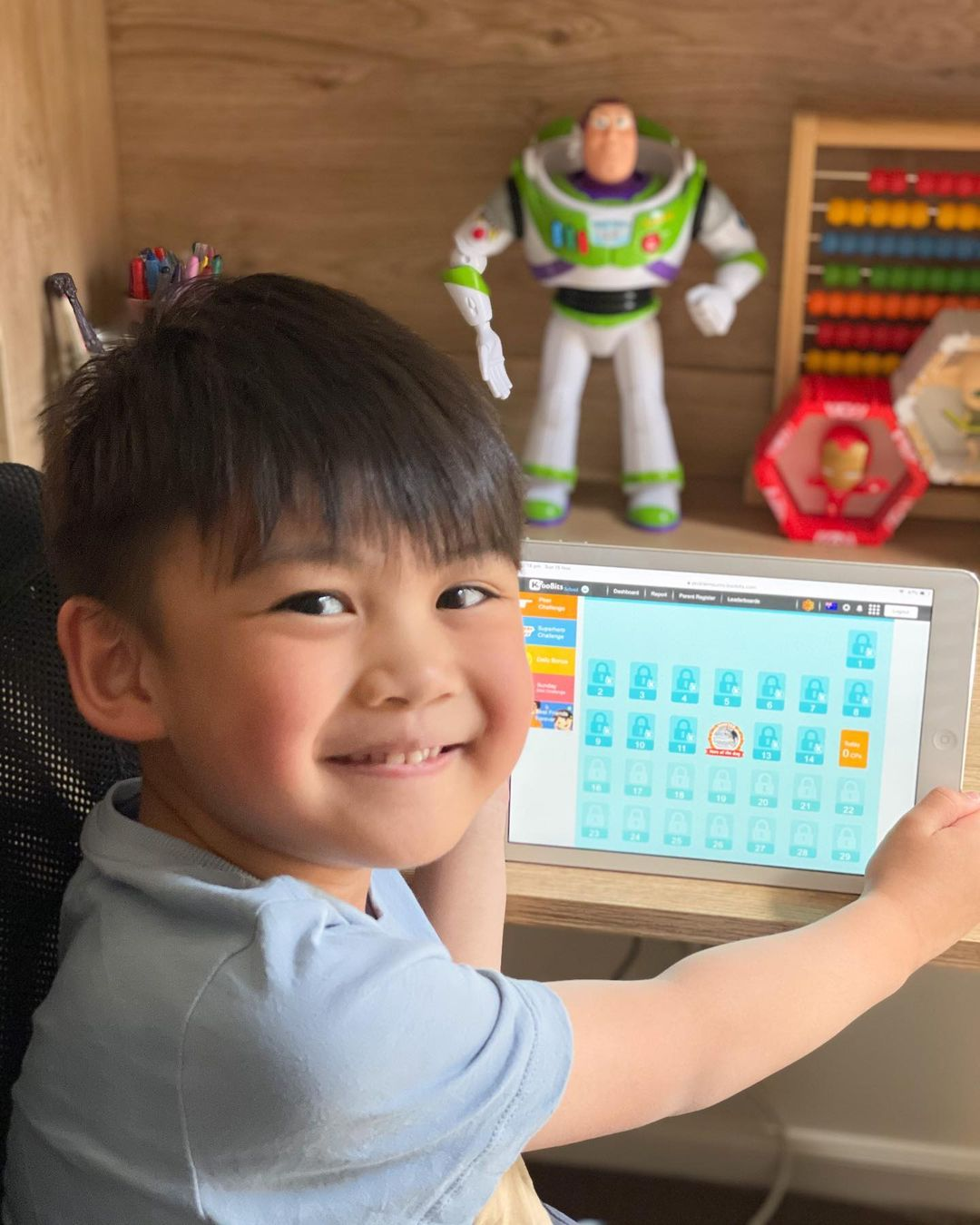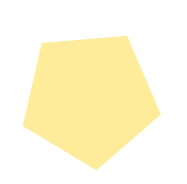## Primary 1 Maths Curriculum

Understanding the Primary 1 Maths Syllabus & How You Can Support Your P1 Child

Stepping into Primary 1 is a huge milestone for any child. It’s also important for parents because the first year lays the foundation for upper primary maths.

To help your child build a strong maths foundation, KooBits’ learning plan gives you all the primary 1 topics taught in Singapore MOE’s syllabus, a globally-recognised curriculum.

We have 100,000+ P1-P6 questions to help your child master the syllabus at their own pace. Start your trial today to get your child learning and practising maths ↓### Numbers Up to 10

1. Count to 10 in numbers
2. Count to 10 in words
3. To count the number of objects
4. Count to 0
5. Compare the number of objects in two or more sets
6. Compare two numbers within 10
7. Count on to find 1 more
8. Count back to find 1 less
9. Concept of more-than using pictorial representation
10. Concept of less-than using pictorial representation
11. Find ordinal relationship with just-before and just-after
12. Find multiple progression ordinal relationship with greater-than and less-than
13. Find the missing number(s) in a given number pattern

1. Make number bonds for numbers up to 10
2. Use number bonds to add in any order
3. Count on to add within 10
4. Adding the parts to find the total
6. Solve word problems involving addition within 10
7. One-step addition problem involving the total quantity after an event
8. To find total quantity for two characters
9. Addition problem involving two persons with more-than relationship. Find quantity for the 2nd person.

### Subtraction Within 10

1. Subtract by taking away
2. Count back to subtract within 10
3. Count on to subtract within 10
4. Use number bonds to subtract
5. Writing subtraction equations
6. Solve word problems involving subtraction by finding the other part
7. Solve word problems involving subtraction by taking away
8. One-step subtraction problem involving the final quantity after an event
9. Subtraction problem involving two persons with less-than relationship. Find quantity for the 2nd person.
10. One-step subtraction problem to find the age difference between two persons

### 2D Shapes

1. Identify 2D shapes
2. Identify 2D shapes from real objects and pictures
3. Grouping shapes by attributes – sides, corners, sizes and colours
4. Complete patterns with 2D shapes
5. Sum numbers involving shapes of circle, triangle, rectangle and square
6. Identify pattern formations involving shapes of circle, triangle, rectangle and square
7. Identify pattern formations involving shapes in combination of circle, triangle, rectangle and square
8. Sum numbers involving length of objects

### Ordinal Numbers

1. Use first, second, up to tenth
2. Use symbols 1st, 2nd, 3rd, up to 10th
3. Use before, after and between to tell position
4. Use left, right and next to
5. Find the number in the number list (numerical ordinal terms)
6. Find the number in the number list (word ordinal terms)
7. Find and compare two numbers in the number list
8. Find and add two numbers in the number list
9. Find and get the difference from two numbers in the number list

### Numbers Up to 20

1. Count to 20 in numbers
2. Count to 20 in words
3. Make a ten and count on
4. Count the number of objects in a given picture
5. Use place value charts to show numbers to 20
6. Use place value to compare two numbers within 20
7. Use place value to compare three numbers within 20
8. Find a number more than another number
9. Find a number less than another number
10. Concept of more-than using pictorial representation
11. Concept of less-than using pictorial representation
12. Find number just-before or just-after another number
13. Find number greater than or smaller than another number
14. Order numbers within 20 by making number patterns

2. Add two 1-digit numbers by making 10
3. Add 1-digit and 2-digit numbers
4. Solve one-step word problems involving addition
5. One-step addition problem involving the total quantity after an event

### Subtraction Within 20

1. Subtract by counting back
2. Subtract from ones only
3. Subtract to get 10 first
4. Subtract from 10 first
5. Solve one-step word problems involving subtraction
6. One-step subtraction problem involving the final quantity after an event
7. One-step subtraction problem to find the age difference between two persons

### Picture Graphs

1. Interpret data in picture graphs
2. Describe the data presented in a picture graph using ‘most’, ‘least’, ‘greatest’, ‘smallest’, ‘as much as’, and ‘as many as’
3. Find sum using data given in a picture graph
4. Find the sum of particular objects using data given in a picture graph

### Numbers Up to 100

1. Count to 40 in numbers
2. Write numbers to 40 in words
3. Count the number of objects in a given picture
4. Use place value charts to show numbers to 40
5. Compare two numbers within 40
6. Compare three numbers within 40
7. Concept of more-than using pictorial representation
8. Concept of less-than using pictorial representation
9. Find number just-before or just-after another number
10. Find number greater than or smaller than another number
11. Find the missing number given a number pattern
12. Count to 100 in tens
13. Count to 100 in tens and ones
14. Use place value charts to show numbers up to 100
15. Compare numbers within 100 when the tens are different
16. Compare numbers within 100 when the tens are equal
17. Find missing numbers in pattern by adding or subtracting
18. Find number just-before or just-after another number
19. Identify the greatest or the smallest number from a given number list
20. Identify the greatest or the smallest number from a given number list, and find the final value after one addition.
21. Identify the greatest or the smallest number from a given number list, and find the final value after one subtraction
22. Identify the greatest or the smallest number from a given number list and find the difference

### Addition & Subtraction Within 100

3. Subtraction without regrouping
4. Subtraction with regrouping
5. Add three 1-digit numbers within 40
6. One-step addition problem involving the total quantity after an event
7. One-step subtraction problem involving the final quantity after an event
8. One-step subtraction problem to find the age difference between two persons
9. Add ones to 2-digit numbers within 100 without regrouping
10. Add tens to 2-digit numbers within 100
11. Add 2-digit numbers within 100 without regrouping
12. Add 2-digit numbers within 100 with regrouping
13. Subtract ones from 2-digit numbers without regrouping
14. Subtract tens from 2-digit numbers
15. Subtract 2-digit numbers without regrouping
16. Subtract 2-digit numbers with regrouping
17. One-step addition problem involving the total quantity after an event
18. One-step subtraction problem involving the final quantity after an event
19. One-step subtraction problem to find the age difference between two persons

### Length

1. Compare height – tall and short
2. Compare length – long and short
3. Compare the lengths of two items
4. Use the terms tallest, longest, and shortest
5. Compare the lengths of three items
6. Measure length using common objects
7. Measure length using different objects
8. Measure items in units
9. Find the total length of two items
10. Find the difference between two items

### Multiplication

2. Write multiplication sentences
3. Multiplication within 40
4. Solve word problems involving multiplication within 40
5. Find the product of two numbers using the term groups
6. One-step multiplication problem to find quantity for an item for one person

### Division

1. Divide by sharing
2. Divide by grouping
3. Division within 20
4. Solve word problems involving division within 20
5. Find the quotient of two numbers using the term groups
6. One-step division problem to find quantity for item each person gets after sharing equally

### Time

1. Tell time to the hour
2. Tell time to the half hour
3. Appropriateness of events at different times of the day

### Money

1. Recognise coins and notes of different denominations
2. Count amount of money in cents up to \$1
3. Count amount of money in dollars up to \$100
4. Match a coin of one denomination to an equivalent set of coins of another denomination
5. Match a note of one denomination to an equivalent set of notes of another denomination
6. Compare amounts of money
7. Use combination of coins to buy things
8. Solve word problems involving adding and subtracting money in dollars only or in cents only
9. One-step addition problem involving cents
10. One-step addition problem involving dollars
11. One-step subtraction problem to find the amount difference (in dollars) between two personsLet KooBits be your child’s learning buddy in Primary 1.
We have 3 powerful features to help students master maths, while having fun at the same time.

### Videos To Bring P1 Syllabus To Life

Our 1,000+ videos bring boring maths topics to life through exciting animations and colourful visuals. These videos are easy to understand, can be played repeatedly, and are perfect learning tools for a digital-first generation.

Watch and learn anytime, anywhere.
Every video links to a specific P1 maths topic.
No supervision required. Teaches and revises maths for you.

### Master Syllabus Through Habit-Building

KooBits makes learning the P1 syllabus easy by creating a habit-forming environment. We use healthy rewards and motivational cues to encourage students to practice daily.

Promotes self-directed learning.
More efficient than cramming for exams.

Master primary 1 syllabus quickly ↓

### Connect P1 Syllabus To Real-World Problems

We make the syllabus more exciting and relevant by going into the “why” behind maths. There are over 90 hours of educational content, showing students how to use these topics to solve real world problems.

Broaden students’ knowledge of the world.
Helps students to apply the skills learnt under each topic.
Aids long-term memory and recall of syllabus.